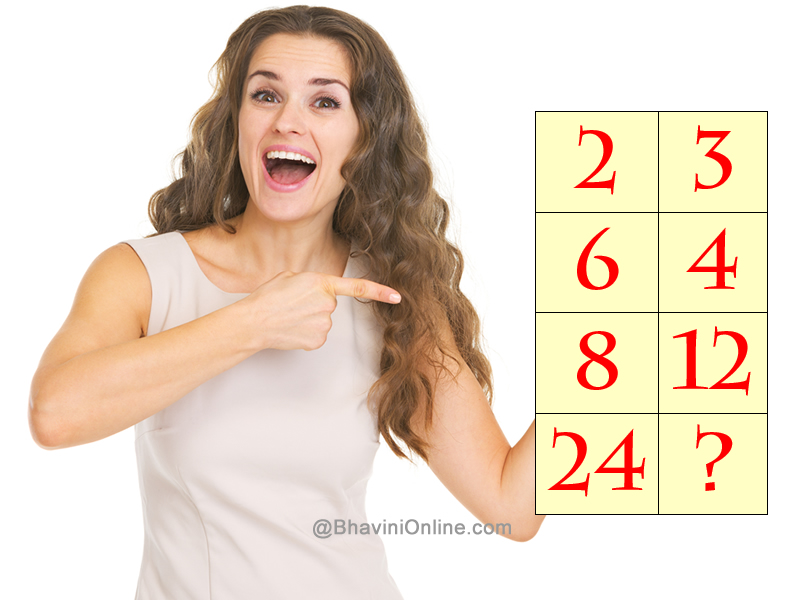# WhatsApp Math Riddle: Find the Missing Number

Look at the table and find the connection between the numbers and then find the missing number.2 3 6 4 8 12 24 ?

If you are able to solve the riddle and get the correct answer share it with your friends on WhatsApp and Facebook.

## 3 Replies to “WhatsApp Math Riddle: Find the Missing Number”

1. Josh says:

36

2. yogi says:

16
2*2=4; 4*2=8; 8*2=16
3*2=6; 6*2=12; 12*2=24

3. Duaj says:

16